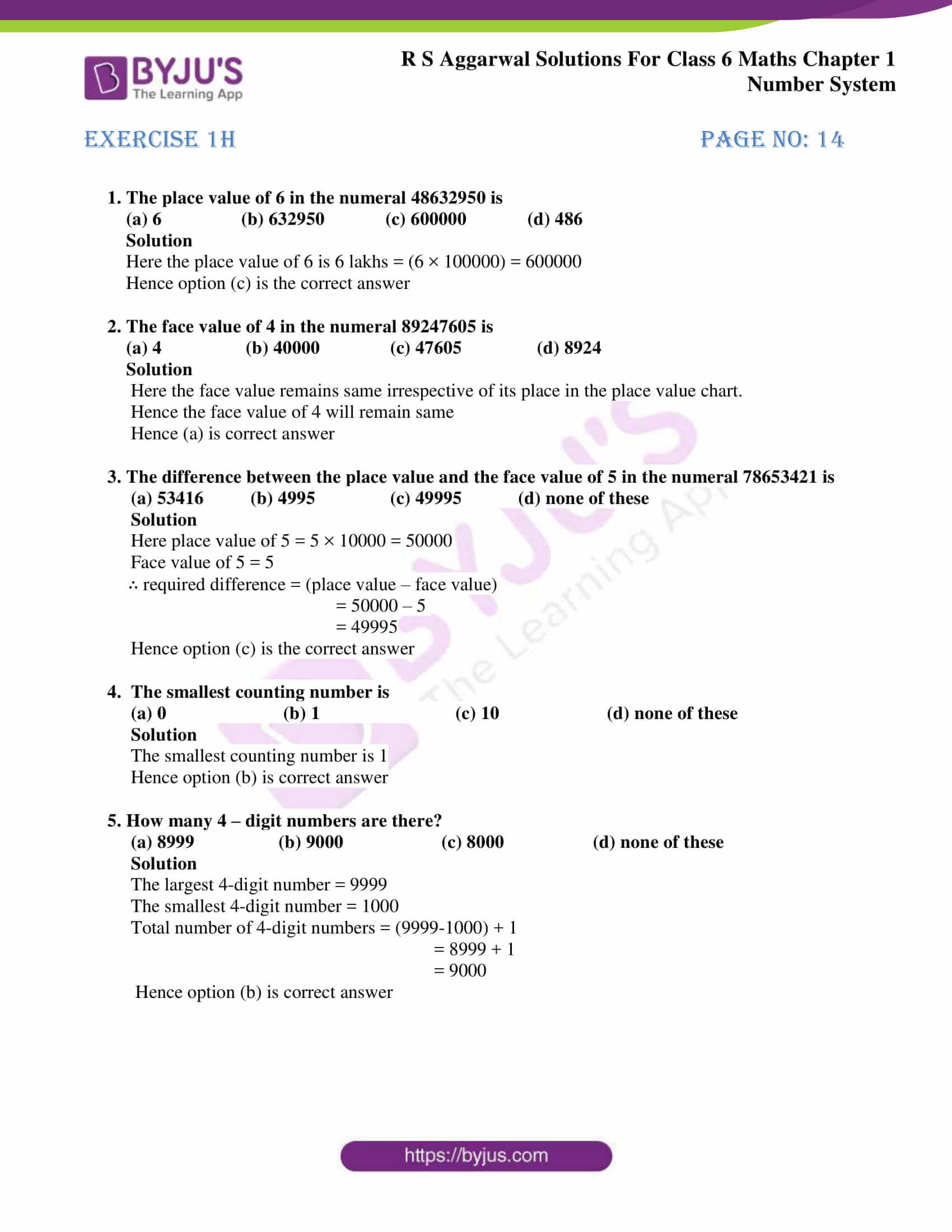# RS Aggarwal Solutions for Class 6 Chapter 1 Number System Exercise 1H

RS Aggarwal Solutions for Class 6, Chapter 1, Number System Exercise 1H are given in PDF format. Students who aspire to understand the chapter in a better way can go through RS Aggarwal Solutions. It will mainly help students understand the concept thoroughly and score high marks in the exams.

RS Aggarwal Solutions are solved with step by step explanation which helps the students in solving problems easily. Exercise 1H of RS Aggarwal textbook has objective type questions which allow the students to analyse their knowledge about the chapter.

## Download PDF of RS Aggarwal Solutions for Class 6 Chapter 1 Number System Exercise 1H### Access answers to Maths RS Aggarwal Solutions for Class 6 Chapter 1 Number System Exercise 1H

1. The place value of 6 in the numeral 48632950 is

(a) 6 (b) 632950 (c) 600000 (d) 486

Solution

Here the place value of 6 is 6 lakhs = (6 × 100000) = 600000

Hence option (c) is the correct answer

2. The face value of 4 in the numeral 89247605 is

(a) 4 (b) 40000 (c) 47605 (d) 8924

Solution

Here the face value remains same irrespective of its place in the place value chart.

Hence the face value of 4 will remain same

3. The difference between the place value and the face value of 5 in the numeral 78653421 is

(a) 53416 (b) 4995 (c) 49995 (d) none of these

Solution

Here place value of 5 = 5 × 10000 = 50000

Face value of 5 = 5

∴ required difference = (place value – face value)

= 50000 – 5

= 49995

Hence option (c) is the correct answer

4. The smallest counting number is

(a) 0 (b) 1 (c) 10 (d) none of these

Solution

The smallest counting number is 1

Hence option (b) is correct answer

5. How many 4 – digit numbers are there?

(a) 8999 (b) 9000 (c) 8000 (d) none of these

Solution

The largest 4-digit number = 9999

The smallest 4-digit number = 1000

Total number of 4-digit numbers = (9999-1000) + 1

= 8999 + 1

= 9000

Hence option (b) is correct answer

## RS Aggarwal Solutions for Class 6 Maths Chapter 1 Number System Exercise 1H

Exercise 1H of RS Aggarwal Solutions for Class 6 Chapter 1 Number System consists of objective type questions with four options containing one right answer. By practising objective type questions, students will come to know the percentage of knowledge they have gained from the chapter. Topics in other exercises are

• International system of numeration
• Comparison of numbers
• Word problems on number operations
• Estimation
• Estimating the products
• Estimating the quotients
• Roman numerals

Students who aim to understand the chapter comprehensively are advised to practice objective type questions given in RS Aggarwal textbook for Class 6 Chapter 1 Number System Exercise 1H.